# Using the GENERATESERIES DAX Function in Power BI

GENERATESERIES is a useful DAX function when you need to create a table with a series of values. Let’s go through how to use it.

In Power BI Desktop, go to the Modeling tab and select New Table: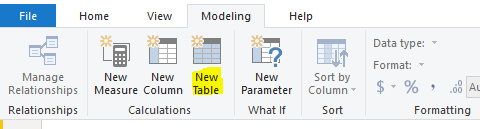Enter GENERATESERIES, and we can see the parameters it takes is:

• StartValue. This is the first row value of our new table.
• EndValue. This is the last row value of our new table.
• IncrementValue. This is the optional value of how much we want to increment. The default is 1.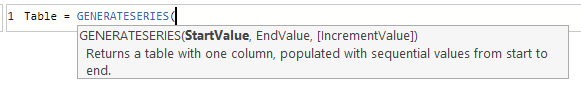For example, if we wanted to generate a table of values from 1 to 100, we can do:

GENERATESERIES(1,100)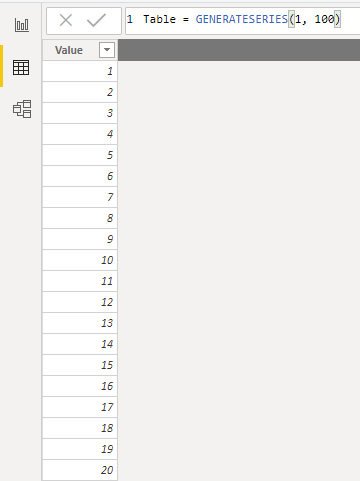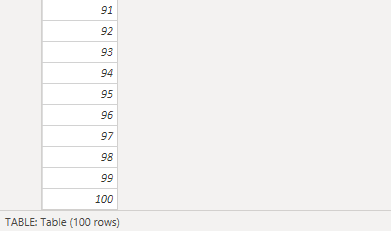If we wanted increments of 9, we could do:

Table = GENERATESERIES(1, 100, 9)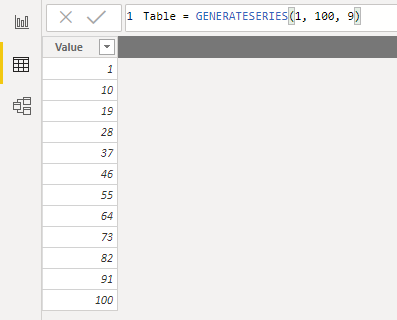Once the table is generated, we can add new columns etc as with any other table.